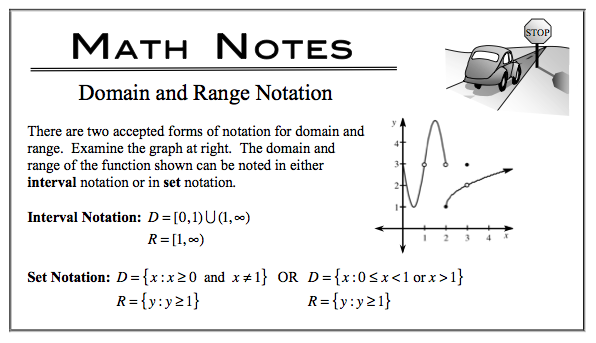### Home > CALC > Chapter 1 > Lesson 1.2.3 > Problem1-53

1-53.

Convert the following domain and range from interval to set notation. Then sketch a possible function.

$D = ( - \infty , 2 ) \cup ( 2 , \infty )$

$R = ( - \infty , - 1 ) \cup ( - 1 , \infty )$The range indicates that this graph never reaches the height of $y = -1$.

The graph of this function could have a hole, jump or vertical asymptote at $y = 2$.